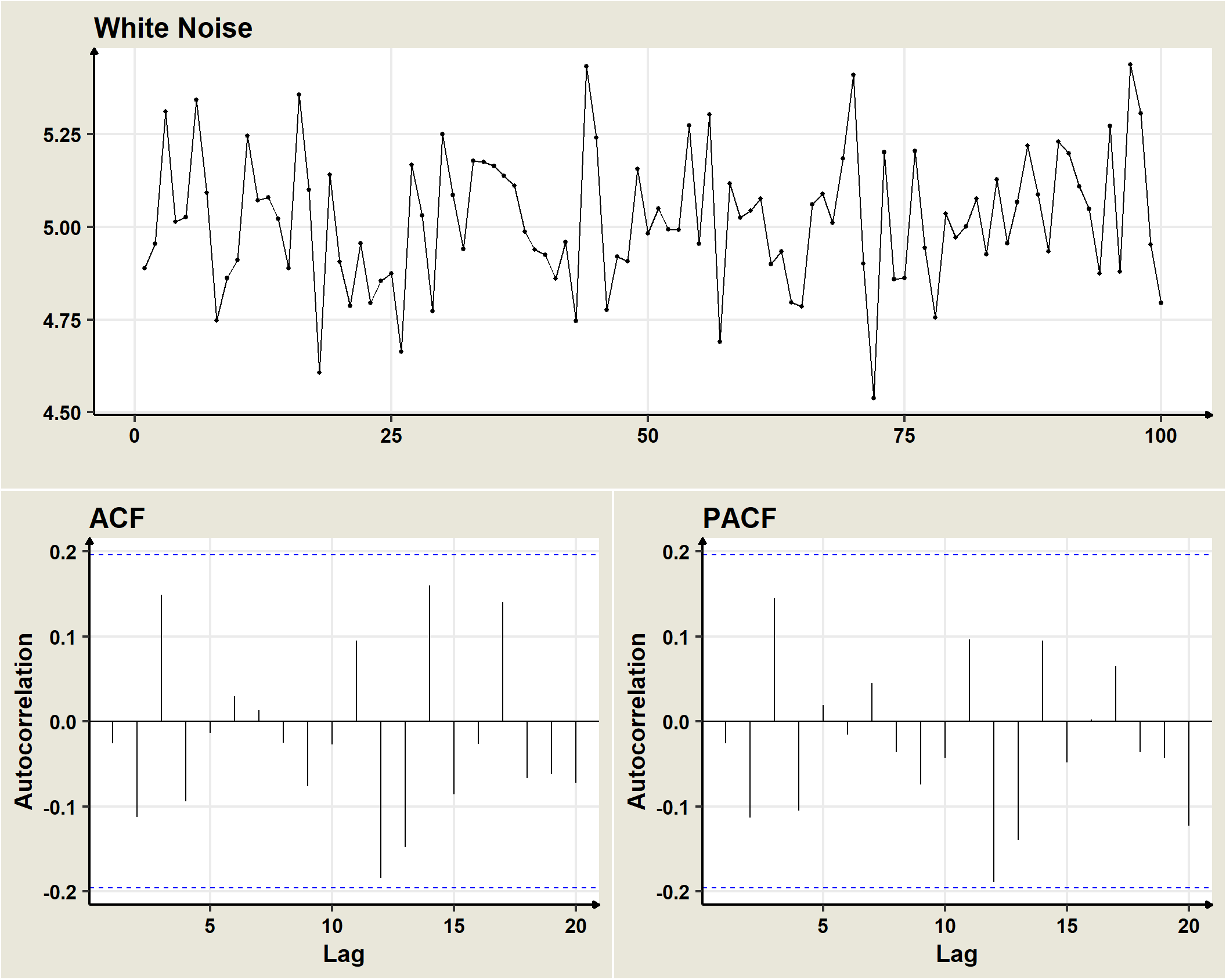## 19.1 Ruido Blanco

White Noise is a stochastic process in which the component random variables are uncorrelated with each other, have mathematical expectation equal to zero and constant variance equal to $$\sigma^2$$.

Gaussian white noises are formed by a succession of random variables with Normal distribution, zero expectation, constant variance and serially uncorrelated with each other. That is, if

$$\omega_t \sim N(0,\sigma^2)$$ is a Gaussian white noise, then $$cov(\omega_t,\omega_{t+k})=0$$In general, a univariate white noise process is a sequence $$(\omega_t)$$ of identically and independently distributed random variables with mean $$0$$ and variance $$\sigma^2_omega$$, which is represented as

$\omega_t \sim IID(0,\sigma^2_\omega)$

Each $$\omega_t$$ follow a Normal distribution, $$(\omega_t)$$ is a Normal or Gaussian White Noise Process.

• We assume that the errors of the processes we will see next are Gaussian white noise, formed by a succession of random variables with Normal distribution, zero expectation, constant variance and serially uncorrelated with each other.

• That is, if $$(\omega_t)$$ is Gaussian white noise, then $$\omega_t \sim N(0,\sigma^2_\omega)$$ and \$cov(t,{t+k})=0.